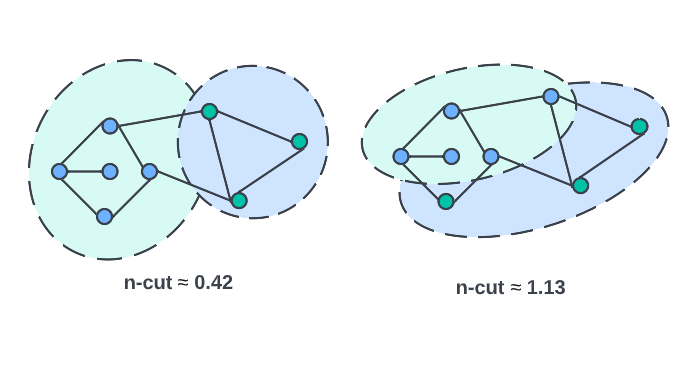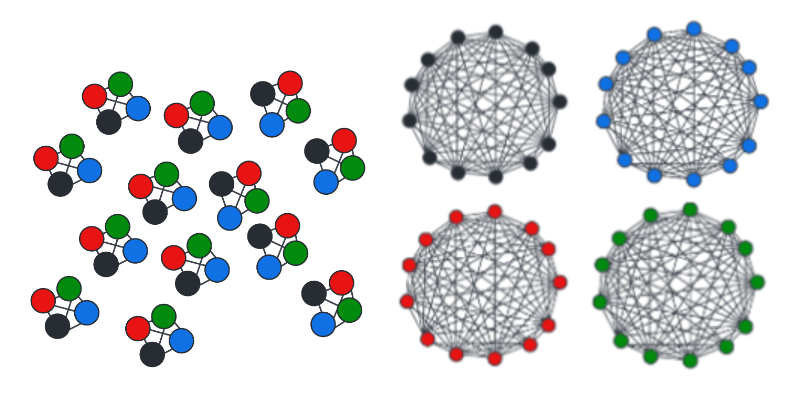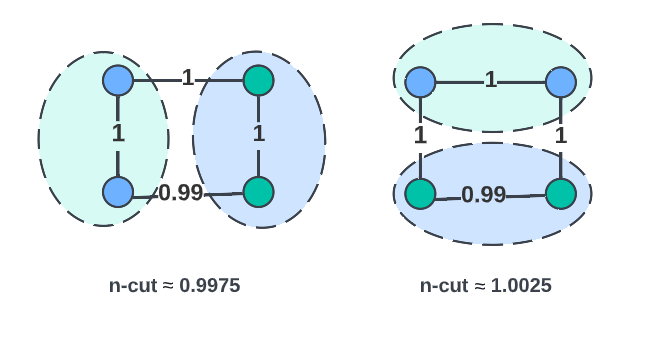# Summary

The Natural Abstraction Hypothesis (NAH) proposes that common high-level abstractions utilised by humans to reason about the world are “natural” and we can expect other intelligent beings to hold similar abstractions. In the project introduction, John Wentworth defined the “Holy Grail'' of the project, a Natural Abstraction Thermometer (NAT), which would enable us to identify the natural abstractions in a system.

Here we look at the easier related problem in terms of modularity and produce a function analogous to NAT in the restricted setting of graph theory. We then use this to gain insight into the relationship between choice, entropy and NAT. We then generalise the process we used to define our analogous function, laying solid foundations for future work.

# Introduction

The Who’s 1969 Rock Classic “Pinball Wizard” describes an arcade prodigy who, despite being both deaf and visually impaired, nonetheless excels at pinball. As Vocalist Pete Townshend puts it:

Ain't got no distractions
Can't hear no buzzers and bells
Don't see no lights a-flashin'
Plays by sense of smell

This of course seems impossible.  But is the situation really so different than if it was a sighted person hitting the bumpers on the machine? A quick calculation shows that there are roughly 10^24 atoms inside a standard issue steel pinball, each with their own position and velocity. The human eye and brain are collecting almost none of that information, yet, we can somehow play pinball, a testament to how useful abstraction is.

The fact that we can understand the pinball's behaviour from only a few parameters (velocity, diameter) is an example of a useful abstraction. The fact that we can group the molecules comprising the ball together, but separate them from the rest of the machine, is an example of modularity.

Modularity has been highlighted as a promising avenue of research for understanding neural networks and will hopefully be applicable to the internal logical structure of anything we would call an AGI. It’s surprising to see that there still isn’t an understanding of what specific definition (or family of definitions) of modularity we should use. Popular definitions of modularity, such as the n-cut metric (described below) require a notion of scale. We hope to find a notion which is “scale-free”.

One approach to find the “natural” definition is to find what seems like a very general measure of modularity in the simplest case (a unweighted unidirectional graph) and then attempt to generalise this to a neural network. If this is successful it would be strong support for the NAH.

# N-Cut

As a starting point we choose an existing modularity function. We assume that if a “natural” notion of modularity exists, functions that can be derived from it would already exist and be in use throughout the literature. We have chosen the normalised cut metric (n-cut) as it is widespread.

We assume the reader is familiar with weighted graphs. Recall that a graph partition is a way of dividing a graph into subgraphs such that no two subgraphs overlap but all the subgraphs combined are equal. In plain english, it’s a way of cutting a graph into pieces and attaching a label to each of those pieces. A specific partition P can be written {X1, X2,...,Xn}, where each Xi is a different subgraph. For a particular Xi, W(edges out of Xi) is the sum of the weights of every edge from a node in Xi to elsewhere in the graph, W(total edges in and out of Xi) is the sum of all edges associated with Xi. Note that the weight of edges that start and end in xi are counted twice.

Then the n-cut metric can informally defined as

The N-cut metric is lower when each subgraph created by a partition has few connections to other subgraphs, compared to its total number of internal connections.

In the case of an unweighted graph, calculating n-cut boils down to counting edges. Here are two example partitions of a simple graph and the resulting value of the n-cut for each.# Comparisons Across Scales

In the simple example above we compared partitions that cut our graph into the same number of pieces, 2. We call a partition that cuts our graph into k pieces an order k partition. If you cut up a cake for 12 guests, then you have made an order 12 partition of the cake, with each slice corresponding to a subgraph.

The n-cut works great when we want to compare two partitions of the same order, but we would like to be able to compare partitions that cut our graph up into different numbers of pieces. The intuition being that finding the “natural modules” of a graph corresponds to finding the partition at which the graph is most modular across all possible partitions, not just ones of the same order. A measure of the modularity of a partition that can be meaningfully compared across orders is said to be scale-free.

Let us quickly demonstrate how the n-cut metric does not satisfy this condition.

Take a standard deck of 52 playing cards and view it as a graph of the relationships between each card. If two cards share a suit, then there is an edge between them. If two cards share a face value, then place an edge between them. To keep this example simple, we don’t worry about any edge for two cards sharing consecutive numeric values. There is 1 edge between the Five of Hearts and the Five of Clubs. There is one edge between the Five of Hearts and the Four of Hearts, but there is no edge between the Five of Hearts and the Four of Clubs. (For the mathematically inclined, we could view this as the Cartesian Product of a fully connected graph with 4 nodes and a fully connected graph with 13 nodes.)

It sure would be nice if this measure corresponded to our natural intuition that the deck naturally divides up into matching suits or matching face values. Consider finding the lowest n-cut metric for both order 13 partitions and order 4. This corresponds into breaking the deck up into either suits or face value and results in the following collections of subgraphs. Note that we have not included edges between subgraphs in these illustrations.If we calculate the n-cut metric for partitioning out deck by card values, we get 10.4 while if we partition the deck by suits we get 0.2.

If we were to naively compare the value of these numbers we might conclude the deck is “more modular” when divided into 4 groups. Let me put that notion to rest pretty quickly. The best partition for dividing the deck in 2 gives a value of 0.26 and the n-cut for the trivial partition (one group) is 0. Unfortunately, these are two completely different orders of partitions and there appears to be no way to meaningfully compare these numbers.

# Choice, A Natural Notion?

So the n-cut metric isn’t going to work which isn’t surprising. It was never intended to provide comparisons between partitions of different orders. So why did we bring it up in the first place?

Let's look at what happens when we go about dividing a deck in 2. The lowest n cut value is achieved by grouping suits. That is all hearts and spades in one pile, diamonds and clubs in the other.

Notice that I've made an arbitrary choice as to which suits to group. In fact there’s 3 different ways (ignoring order) that I could have decided to split the deck in half. To put it another way, there are 3 completely different partitions that all achieve the lowest n-cut value for partitions of order 2. Compare this to the above case of the finding the optimal way to split the deck into 4. Exactly 1 partition achieves the lowest n-cut value out of all possible order 4 partitions.

This leads us to the definition of a natural module number.

An unweighted undirected graph has a “natural module number” of k when there is strictly one minimum partition of order k.

To put this another way, specifying just the number k uniquely specifies the partition you mean. In the case of the deck of cards the natural modules numbers correspond to be {1,4,13,52}.

This gives us a definition of the Natural Modularity Function, M for undirected graphs. This is a function that takes a graph G as input and spits out a set of integers, the Natural Module Numbers. If you find the partition with the lowest n-cut metric for an order corresponding to one of the natural module numbers, then you have identified the The Natural Modules.

Let's try and justify why this notion of choice might be appropriate. Firstly, let's state the obvious that choice here is clearly related to information and entropy. If someone wishes to communicate a partition that doesn’t match one of the natural module numbers, they need to specify how many partitions to divide our graph into and then transmit additional information about specifics of that partition. In the worst case (there are no connections between nodes) specifying the exact partition involves specifying an amount of information proportional to the logarithm of The Bell Number.

When specifying the modules which are the natural modules, only a small amount of information is needed (which of the natural module numbers you have chosen).

# Our Actual Goal

Lets now pivot to how we can extend M (The Natural Modularity Function) from undirected graphs to a more general setting.

We would ideally like a comprehensive list of requirements for M, but no such list exists. Instead we assume some very general things about the function and hope that we will be able to gain further insight late on.

At minimum we would like M to:

1. Be scale free
That is it doesn't require you to specify the size of clusters its looking for or number of partitions needed. It’s purely a function of the object we are investigating.

2. Readily generalises to the various types of structures we expect to be modular
Obviously coming up with a definition that doesn’t translate easily from graphs would not be very useful.
3. Capture the relationship between modularity and transmitting information
Just like we saw in the simple graph framework above.

# General Method for Finding M

You are trying to make your way up a mountain, after a little planning you find a slope that looks relatively clear of trees and debris from the bottom. That doesn’t mean it is the best path, but at least it’s a plan. In the worst case scenario when you get stuck halfway and must return to the ground, you will at least learn some valuable information help plan the next attempt.

Our chosen road is as follows. It is by no means the only way of solving this problem. The hope that if it is not successful and understanding of why it is not successful may lead to a better approach. We’ve already covered steps 1 and 2 in this document.

1. Pick an already existing scaled modularity function that applies to undirected unweighted graphs.
2. Extend it to a scale free version.
3. Generalise it to weighted graphs.
4. Generalise it to weighted and directed graphs.
5. Generalise it to more complicated domains.

Note that we are open to the possibility that n-cut was not the right metric to start with, but the notion of choice we outlined to extend it to a scale free version should hold for other metrics also.

# Extension to Weighted Graphs

The good news is n-cuts extends very easily to weighted graphs. The bad news is the above notion of discrete “choice” requires some refinement.

Extension to weight graph has faced the hurdle that two almost equivalent partitions may not have equal n-cut values. This means if we strictly define our module numbers the same way we can get graphs which have a single minima for graph cut metric for some number of partitions but do not have a clear sense of modularity. To demonstrate this, compare the following two graph partitions.Using the definition of M we used in the case of unweighted graphs, we would have to consider the lowest n-cut to be a natural partition. This is obviously not a good definition A correct definition should be immune to “noise” in the weights if it is useful for communication between parties.

The exact solution to this is as yet unclear but we have a good understanding of the problem. We first need to find the correct notion of specifying a notion of “distance” between partitions such that we can then distinguish good partition choices which correspond to “close” or “distant” partition choices. Our problem is not finding a way to do it, but finding the right way, with at least 5 different metrics that might be appropriate.

Secondly, we need a way of explicitly comparing n-cut values when they are “almost” equal.

From there it’s a matter of finding the appropriate mathematical machinery to capture the “choice/entropy” notion we found in discrete graphs.

# 15

New Comment

I am very happy you're looking into this. I've never seen a well motivated procedure for choosing k, and we're going to need one.

Let's try and justify why this notion of choice might be appropriate. Firstly, let's state the obvious that choice here is clearly related to information and entropy. If someone wishes to communicate a partition that doesn’t match one of the natural module numbers, they need to specify how many partitions to divide our graph into and then transmit additional information about specifics of that partition. In the worst case (there are no connections between nodes) specifying the exact partition involves specifying an amount of information proportional to the logarithm of The Bell Number.

This alone doesn't seem satisfactory to me. I think you could make up lots of other prescriptions for picking partitions that take little information to specify.  E.g.:  "The ones with minimal n-cuts for their k that come closest to having equal numbers of nodes in each subgraph."

"Cuts with a single minimum n-cut partition" could be what we're looking for, but I don't see anything yet showing it has to be this, and it could not be anything else.

We'll probably have a post with our own thoughts on measuring modularity out soon, though it'll be more focused on how to translate a neural network into something you can get a meaningful n-cut-like measure that captures what we care about for at all.

If you're interested in exchanging notes, drop me or TheMcDouglas a pm.

IMO ncut divided by the number of clusters is sort of natural and scale-free - it's the proportion of edge stubs that lead you out of a randomly chosen cluster. Proof in appendix A.1 of Clusterability in Neural Networks

The first thing that comes to my mind is to consider probability distributions over partitions, and find the lowest-valued one of those. The weights could be interpreted as credences that a given partition is in fact "the correct one". After all, I doubt we have a specific idea of the boundary between two given objects - we can't map which atom is part of which object, even if we had microscopic vision and could see the atoms. The border is fuzzy. Seems like a distribution over partitions would thus do better than a single partition.

Ah, but then the problem is, how do you score them? Hmm. I may need to think about this. It's the kind of mathematical puzzle I like playing with.